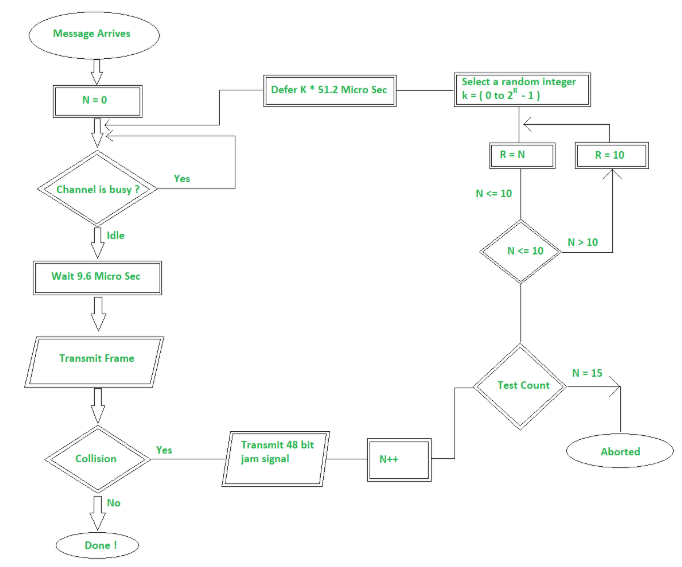Open in App
Not now

# Implementation of a Back-off Algorithm for CSMA/CD

• Difficulty Level : Hard
• Last Updated : 05 Jul, 2022

Prerequisite – Back-off Algorithm for CSMA/CD
Ethernet network may be used to provide shared access by a group of attached nodes to the physical medium which connects the nodes. These nodes are said to form a Collision Domain. When there is data waiting to be sent, each transmitting NIC also monitors its own transmission. If it observes a collision, it stops transmission immediately and instead transmits a 48-bit jam sequence.

Flow chart of the transmit procedure is shown below.Flow Chart – Back-off

The transmitter initializes the number of transmissions of the current frame (N) to zero and starts listening to the cable to see if any message bits are travelling. If the cable is not idle, it waits (Defers) until the cable is idle. After the carrier being Idle it waits for more than 9.6 microseconds to allow the nodes to prepare themselves for the transmission that is about to be happen next. This is done to avoid starvation.

• Transmit Frame :
It transmit the frame through the cable and if no collision occurs then the message is sent successfully. If there is a collision as the message frame will come back to you, then every station on the way who detects the collision should emit a jamming signal so that every detected stations should abort sending a signal anymore.

• Jamming Signal :
Different type of frequency is reserved for jamming signal. It is sent in order to stop all the stations to discard the packets which you’ve accepted. After seeing the jamming signal the sender abort sending the packet.

After collision, the collision number if is increased (N++) as you can in the above flow chart.

• Test Count :
If the network is busy, a re-transmission may still collide with some other re-transmitted frame (or possibly new frames being sent for the first time by another NIC). So the test count counts the number of re-transmission attempts using the variable N and attempts to re-transmit the same frame upto 15 times. After 15, it gets aborted.

Note :
The value of N varies from LAN to LAN. It is implementation dependent.

If the value of N is less than 15, then it will check if the value of N is less than 10. If less than 10 then the variable R will be set same as N but if the value of N is greater than 10 then the value of R will be set to 10. This gives an idea that the value of R is limited till 10.

• Select Random :
A random integer is selected from the range of (0 to 2R-1). Then a waiting time (K * 51.2) is evaluated. Here 51.2 is a slot time(Tslot) given to a station. Again it is implementation dependent it varies from LAN to LAN depending on what the bandwidth is.
After it, again the whole procedure is repeated.

Example :
Suppose 2 stations A and B start transmitting data at the same time then, collision occurs. After two collision, N = 2, Therefore R = 2, and the set is {0, 1, 2, 3} giving a one in four chance of collision. This corresponds to a wait selected from {0, 51.2, 102.4, 153.6} microseconds.

After 3 collisions, N = 3, Therefore R = 3, and the set is {0, 1, 2, 3, 4, 5, 6, 7}, that is a one in eight chance of collision.

But after 4 collisions, N=4, Therefore the set becomes {0, 1, 2, 3, 4, 5, 6, 7, 8, 9, 10, 11, 12, 13, 14, 15}, that is a one in 16 chance of collision. The scaling is performed by multiplication and is known as exponential back-off. That is how CSMA/CD scale to large numbers of NICs – even when collisions may occur. The algorithm includes a threshold of 1024. That’s for a ten times collision as even if the value of N is increasing till 15 we’re setting the value of R as 10 and if the number of collision is exceeding till 15 then it is aborted. The reason is that with the number of increase in collision the number of NICs required is more and the period of station to be deferred is large. Since a set of numbers {0, 1, …, 1023} is a large set of numbers, there is very little advantage from further increasing the set size.

Each time the transmission is occurring the number of re-transmission of a single frame is limited till 16 attempt ( N = 15 ). After this the frame is discarded. But in practice, a network unless or until it is not overloaded is not allowed to discard the packet in this way.

Explanation :
When a frame is transmitted for the first time- Reset : N = 0.

If there was a collision, the first re-transmission attempt will use N = N + 1 = 0 + 1 = 1, R = 1 and the node will pick a random number K from the range of { 0, 1 }.

If the node is facing another collision while transmitting the same frame then N = N + 1 = 2, R = 2 and the node will pick a random number K from the range of { 0, 1, 2, 3 }.

And so on…

My Personal Notes arrow_drop_up